### High Reflectors

 High reflectors are optical coatings maximizing the reflectance of an optical surface in a specified spectral range. The coating performance outside of the specified spectral range is generally of no interest. High reflectors can be divided into two groups: metal coatings and dielectric coatings. Thin layers of silver, aluminum, gold provide high reflectance in a wide spectral range but they are extremely fragile and it is difficult to maintain them over long periods of time. Often metal high reflectors require a protective dielectric layer. Dielectric high reflectors can be considered as an alternative but they exhibit much narrower high reflection range. OptiLayer provides various numerical tools for designing dielectric and metal-dielectric high reflectors. Reflectance of a high reflector calculated with the help of the needle optimization technique.Watch a video example on YouTube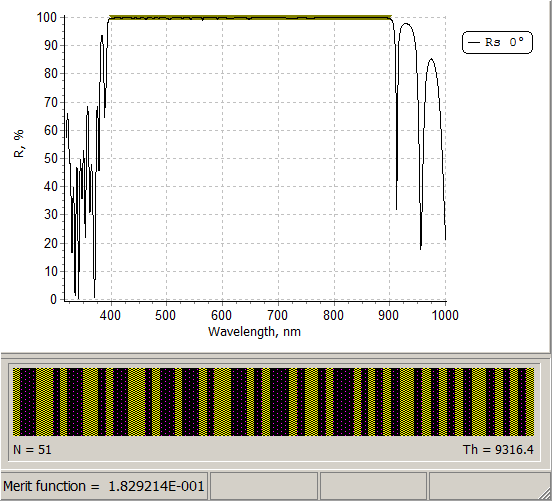Refractive index profile of a high reflector calculated with the help of the needle optimization technique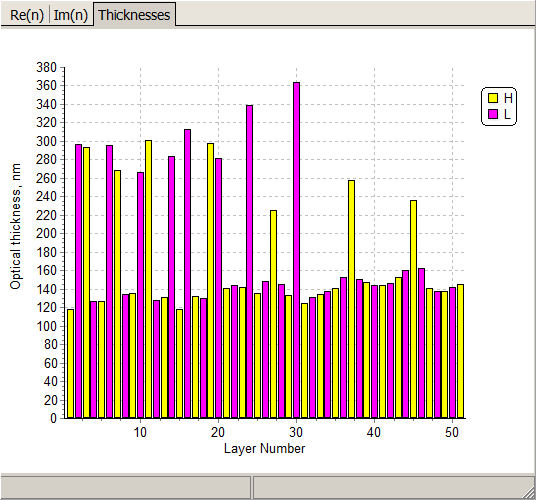Refractive index profile of a high reflector calculated with the help of the formula constrained optimization option of OptiLayer. We can observe a combination of three near quarter wave stacks.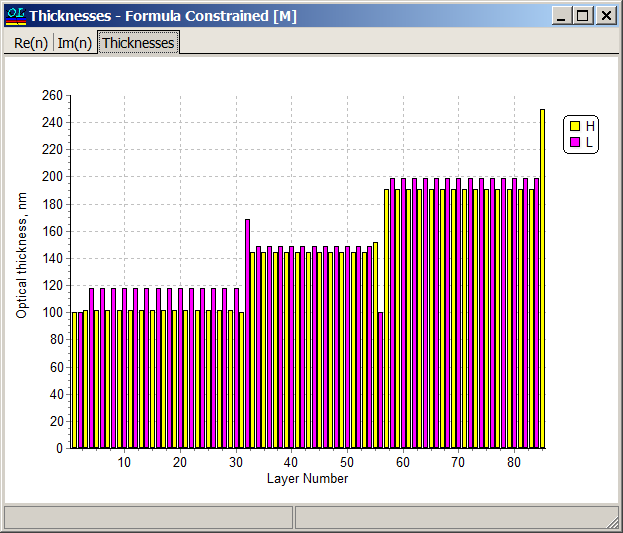Reflectance of a high reflector calculated with the help of the formula constrained optimization option of OptiLayer. See the details at the next page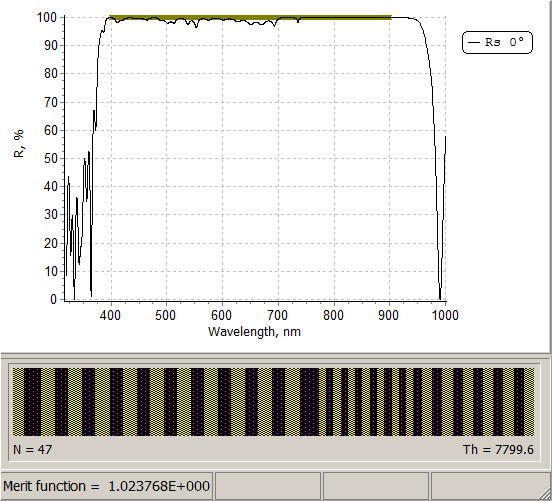### Formula Constrained Optimization

Formula Constrained Optimization is a specialized optimization option based on formula representation of a multilayer coating. Some formula parameters may be considered as unknown parameters, part of which are continuous variables changing within some limits, other parameters may be integer parameters having a predefined set of values.

 For example, a quarter-wave stack with n pairs of layers with another non-quarter wave stack with m pairs and QWOT thicknesses equal to a and b can be represented as $(HL)^n\; (aHbL)^m$ We can consider some set of possible values for integers n and m, and for every possible combination of n and m OptiLayer will try to find optimal values of continuous parameters a and b, optimizing the design performance with respect to loaded targets. Another example may be a stack having period with varying optical thicknesses. Ultra-wide range high reflectors, chirp mirrors and other coatings can be designed in this way. Example. High reflector operating in the spectral range from 400 nm to 900 nm. Refractive indices of layer materials nH=2.35 and nL=1.45, glass substrate. Width of the first high reflection zone of a quarter wave mirror with the central wavelengthcan be estimated with the help of a known formula: $\Delta= \lambda_u-\lambda_l, \;\; \frac{\lambda_u}{\lambda_l}=\frac{\pi+\arccos(-\xi)}{\pi-\arccos(-\xi)}$ $\xi=\frac{n_H^2+n_L^2-6n_H n_L}{(n_H+n_L)^2}$ where $$\lambda_u$$ and $$\lambda_l$$ are upper and lower boundaries of the high reflection zone. If the central wavelength is 650 nm then the width of the high reflection zone of a quarter wave mirror is about 200 nm. In our design problem we need to cover a spectral range 400-900 nm, i.e. we need a high reflection zone of 500 nm. It means that we need to combine several quarter wave or near quarter wave stacks.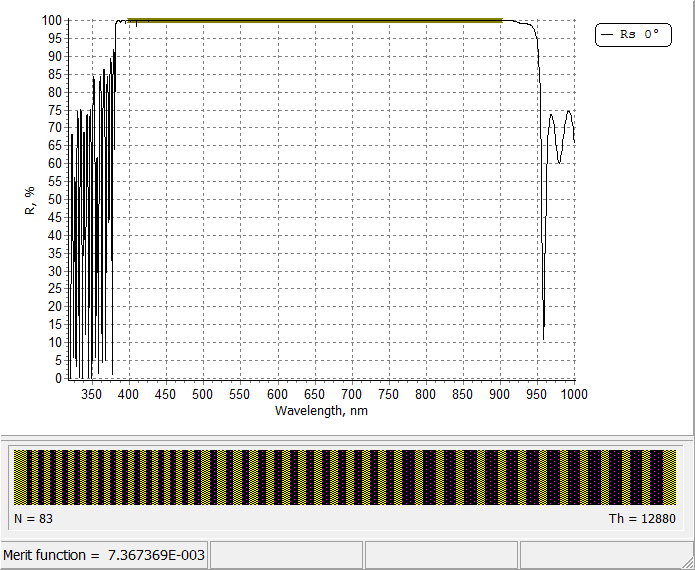Illustration of the design process of a wide band reflector. In order to observe the design process, put the mouse of the picture.Assuming that $$\lambda_l=400$$nm and using formulas above, it is possible to estimate that three quarter wave stacks are required. The corresponding width of the high reflection zones are: $\Delta_1=140\; nm,\;\Delta_2=200\;nm,\;\Delta_3=260\;nm$    Then we need three quarter wave or near quarter wave stacks and matching layers.  The design can be therefore represented by a formula: m1, m2, m3 are integer parameters indicating the number of pairs  a1, b1,... are continuous parameters expressing the fraction of quarter wave optical thickness. m1, m2, m3 can, for example, take values from 5 to 14. The parameters a1, b2,... can be varied in the segments [0.8;2] $c_1 H d_1 L (a_1H b_1 L)^{m_1}\;\; c_2Hd_2L (a_2Hb_2L)^{m_2} c_3Hd_3L (a_3H b_3 L)^{m_3} c_4 H$ In OptiLayer design formulas and their parameters can be specified in Synthesis --> Formula Constrained Optimization: OptiLayer will consider all possible combinations of specified integer parameters m1, m2, m3. Each of these parameters can take 10 values: 5, 6, ..., 14. For each m1, m2, m3 combination, OptiLayer optimizes the design with respect to continuous parameters a1, b1, c1,... All calculated design are stored in the "Design Collection Window". The designs are ranged with respect to the merit function values.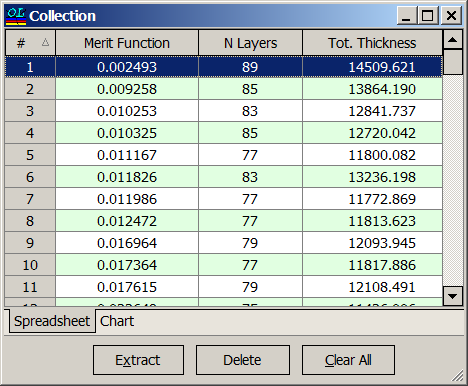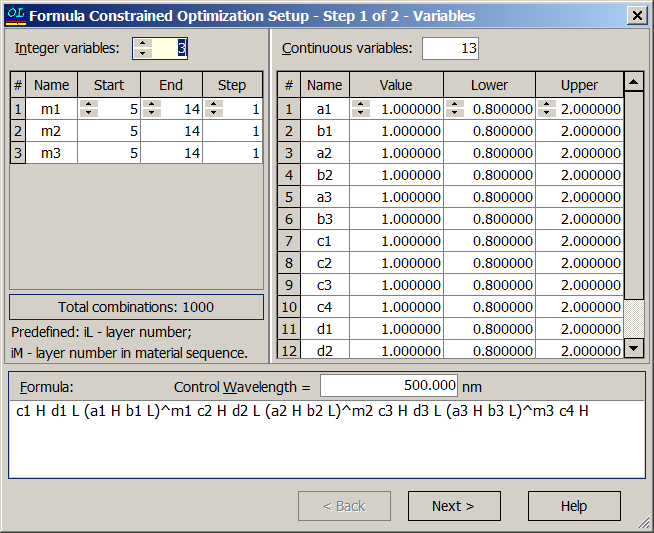This design example is illustrated in our video example "High Reflector Part II" on YouTube

### Easy to startOptiLayer provides user-friendly interface and a variety of examples allowing even a beginner to effectively start to design and characterize optical coatings.        Read more...

### Docs / SupportComprehensive manual in PDF format and e-mail support help you at each step of your work with OptiLayer.If you are already an experienced user, OptiLayer gives your almost unlimited opportunities in solving all problems arising in design-production chain. Visit our publications page and challenge page.